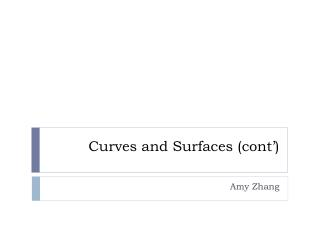DownloadDownload PresentationCurves and Surfaces (cont’)

# Curves and Surfaces (cont’)

Télécharger la présentation## Curves and Surfaces (cont’)

- - - - - - - - - - - - - - - - - - - - - - - - - - - E N D - - - - - - - - - - - - - - - - - - - - - - - - - - -
##### Presentation Transcript

1. Curves and Surfaces (cont’) Amy Zhang

2. Conversion between Representations • Example: Convert a curve from a cubic B-spline curve to the Bézier form:

3. From Curve to Surface • Recall that a parametrically defined curve in 3D is given by three univariate functions: • where 0 ≤u≤1 • By varying u from 0 to 1, a curve is swept out in 3D • Similarly, a parametric surface is defined by three bivariate functions: • where 0 ≤u≤1 and 0 ≤v≤1 • By varying u from 0 to 1while keeping v constant, a curve is swept out in 3D • An infinity of such curves exists as v is varied from 0 to 1, and this defines a surface in 3D

4. Parametric Surfaces • A single surface element, also known as a patch, is the surface traced out as the parameters (u,v) take all values between 0 and 1 • Complex surfaces are modelled using nets of individual patches analogous to the way in which complex curves are made up of individual curve segments The teapot is made up of 32 Bézier patches

5. Like in the case of curves, cubic basis functions, which are a good compromise between complexity and flexibility, are most commonly used in compute graphics • Bicubic parametric patches are defined over a rectangular domain in u,v-space and the boundary curves of the patch are themselves cubic polynomial curves

6. Parametric Surfaces • Advantages of patch representation over polygon mesh representation: • Conciseness: A parametric representation of a surface is “exact” and “economic”, and mass properties, like surface area and volume, are extractable from such a description

7. Parametric Surfaces • Deformation and shape change: Deformation of a parametric surface can be achieved by moving the control points that define it. The deformations will appear just as smooth and accurately represented as the un-deformed counterpart when rendered

8. Bicubic Surfaces • A bicubic parametric patch can be expressed in terms of the basis function and the control points: • where pij is a set of 16 control points: • The net of control points forms a polyhedron in cartesian space and the position of the points in this space control shape of the surface

9. Bicubic Surfaces • Example: The effect of lifting one of the control point of a Bézier patch

10. Bicubic Surfaces • Matrix formulation: • where • The matrix M is the characteristic matrix of the particular form e.g.,MB for Bézier patch and MBS for B-spline patch • The matrix P is a 4×4 matrix that contains the 16 control points

11. Bézier Patch • A Bézier patch is defined in matrix notion by: • and alternatively by: • Note that the boundary curves of a Bézier patch are themselves Bézier curves, e.g., • The Bézier patch has analogous properties to and advantages of the Bézier curve

12. Bézier Patch • Differentiation:

13. Bézier Patch • Differentiation at the corners:

14. Bézier Patch • Joining 2 Bézier patches Q and R with control points qij and rij • Zero order continuity: q3i= r0i for i =0,…,3 • First order continuity:(q3i–q2i) =k(r1i–r0i)for i =0,…,3 • The above constraints may be too severe: Any corner point cannot be moved without controlling 8 adjacent points to maintain continuity

15. Bézier Patch • Recall that a Bézier curve can be rendered efficiently by recursive subdivision

16. Bézier Patch • Similarly, a Bézier Patch can be rendered by successively subdividing the control polygons into a series of polygons that represent the Bézier surface to a given tolerance in curvature. • The process of recursive subdivision of a Bézier Patch is called patch splitting. • The result polygons from patch splitting can be passed to a conventional polygon render and standard rendering techniques can be used to render surfaces made up of nets of patches.

17. Bézier Patch • Due to the orthogonality of the parametric directions u& v, a Bézier patch can be split in one parameter without considering the other

18. Bézier Patch • Consider a patch to be made up of 4 curves, the control points of which correspond to the rows / columns of P • Splitting is then applied to these curves separately, yielding 2 sets of 4 curves qij& rij • These 2 sets of 4 curves then give 2 subpatches of the original patch

19. Bézier Patch • A given patch is subdivided down until the convex hull is deem to be sufficiently planar and the edges are sufficiently linear: • Flatness is tested by forming a plane between 3 corner control points and measuring how far the remaining control points deviate from this plane • Linearity of the edges is tested by measuring how far the interior control points of the edge deviate from the line joining the end control points

20. Bézier Patch • The patch is then turned into 2 triangles by taking the 4 corners of the patch as the triangle vertices • The surface normal at each corner is then computed as the cross product of the 2 tangent vectors at the corner, e.g.,

21. Bézier Patch • The cracking problem will appear due to the independent subdivision of adjacent subpatches:

22. Uniform B-spline Patch • A uniform B-splinepatch is defined in matrix notion by: • A composite B-spline surface is C2 continuous across the patches • The B-spline patch has analogous properties to and advantages of the B-spline curve • Converting a B-spline patch to a Bézier patch for rendering: